libxlsxwriter
Working with Outlines and Grouping

Excel allows you to group rows or columns so that they can be hidden or displayed with a single mouse click. This feature is referred to as Outlines and Grouping.

Outlines can reduce complex data down to a few salient sub-totals or summaries. For example the following is a worksheet with three outlines.Rows 2 to 11 are grouped at level 1 and rows 2 to 5 and 7 to 10 are grouped at level 2. The lines at the left hand side are called "outline level" bars and the level is shown by the small numeral above the outline.

Clicking the minus sign on each of the level 2 outlines will collapse and hide the data as shown below.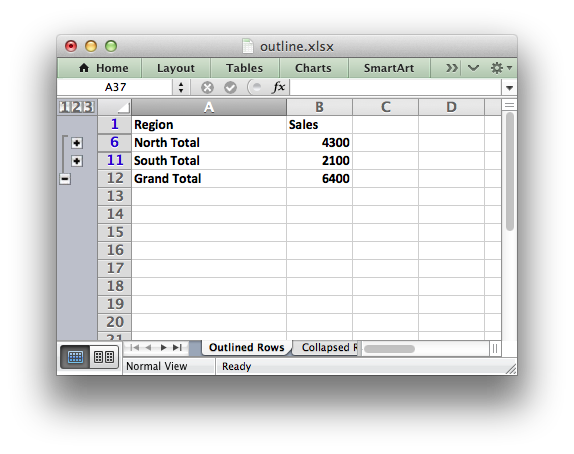The minus sign changes to a plus sign to indicate that the data in the outline is hidden. This shows the usefulness of outlines: with 2 mouse clicks we have reduced the amount of visual data down to 2 sub-totals and a master total.

Finally, clicking on the minus sign on the level 1 outline will collapse the remaining rows as follows: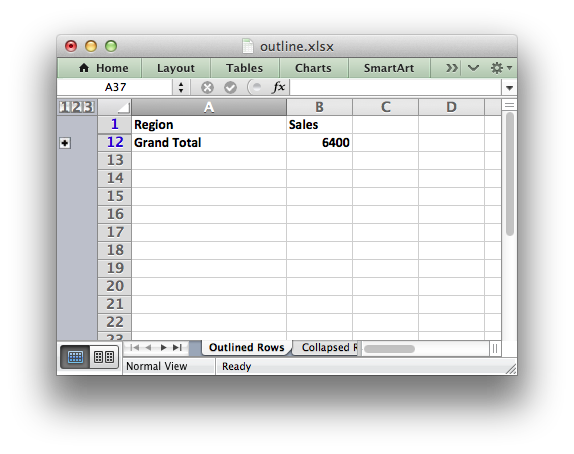# Outlines and Grouping in libxlsxwriter

Grouping in `libxlsxwriter` is achieved by setting the outline level via the `worksheet_set_row_opt()` and `worksheet_set_column_opt()` worksheet functions:

Adjacent row or columns with the same outline level are grouped together into a single outline.

The `options` parameter is a lxw_row_col_options struct. It has the following members:

• ‘'hidden’` -`'level'` -`'collapsed'`

Options can be set as follows, for example to set up an outline for rows:

// The option structs with the outline level set.
lxw_row_col_options options1 = {.hidden = 0, .level = 2, .collapsed = 0};
lxw_row_col_options options2 = {.hidden = 0, .level = 1, .collapsed = 0};
// Set the row options with the outline level.
worksheet_set_row_opt(worksheet, 1, LXW_DEF_ROW_HEIGHT, NULL, &options1);
worksheet_set_row_opt(worksheet, 2, LXW_DEF_ROW_HEIGHT, NULL, &options1);
worksheet_set_row_opt(worksheet, 3, LXW_DEF_ROW_HEIGHT, NULL, &options1);
worksheet_set_row_opt(worksheet, 4, LXW_DEF_ROW_HEIGHT, NULL, &options1);
worksheet_set_row_opt(worksheet, 5, LXW_DEF_ROW_HEIGHT, NULL, &options2);
worksheet_set_row_opt(worksheet, 6, LXW_DEF_ROW_HEIGHT, NULL, &options1);
worksheet_set_row_opt(worksheet, 7, LXW_DEF_ROW_HEIGHT, NULL, &options1);
worksheet_set_row_opt(worksheet, 8, LXW_DEF_ROW_HEIGHT, NULL, &options1);
worksheet_set_row_opt(worksheet, 9, LXW_DEF_ROW_HEIGHT, NULL, &options1);
worksheet_set_row_opt(worksheet, 10, LXW_DEF_ROW_HEIGHT, NULL, &options2);Or an outline for columns:

lxw_row_col_options options1 = {.hidden = 0, .level = 1, .collapsed = 0};
worksheet_set_column_opt(worksheet, COLS("B:G"), 5, NULL, &options1);The following example sets an outline level of 1 for rows 1 to 4 (zero-indexed) and columns B to G. The parameters `height`, `width` and `cell_format` are assigned default values:

lxw_row_col_options options1 = {.hidden = 0, .level = 1, .collapsed = 0};
worksheet_set_row_opt(worksheet, 1, LXW_DEF_ROW_HEIGHT, NULL, &options1);
worksheet_set_row_opt(worksheet, 2, LXW_DEF_ROW_HEIGHT, NULL, &options1);
worksheet_set_row_opt(worksheet, 3, LXW_DEF_ROW_HEIGHT, NULL, &options1);
worksheet_set_row_opt(worksheet, 4, LXW_DEF_ROW_HEIGHT, NULL, &options1);
worksheet_set_column_opt(worksheet, COLS("B:G"), LXW_DEF_COL_WIDTH, NULL, &options);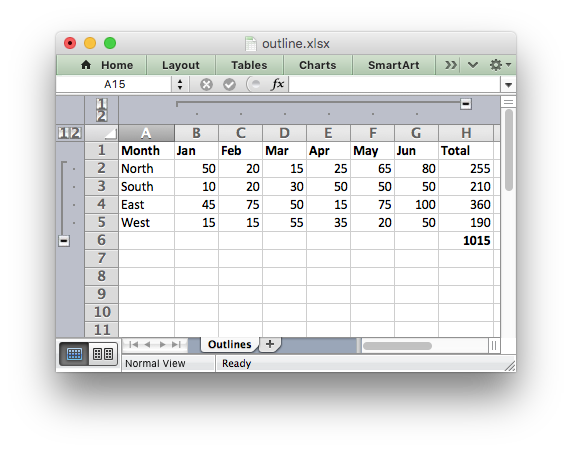Rows and columns can be collapsed by setting the `hidden` member for the hidden rows/columns and setting the `collapsed` member for the row/column that has the collapsed ‘’+'` symbol:

lxw_row_col_options options1 = {.hidden = 1, .level = 1, .collapsed = 0};
lxw_row_col_options options2 = {.hidden = 0, .level = 0, .collapsed = 1};
worksheet_set_row_opt(worksheet, 1, LXW_DEF_ROW_HEIGHT, NULL, &options1);
worksheet_set_row_opt(worksheet, 2, LXW_DEF_ROW_HEIGHT, NULL, &options1);
worksheet_set_row_opt(worksheet, 3, LXW_DEF_ROW_HEIGHT, NULL, &options1);
worksheet_set_row_opt(worksheet, 4, LXW_DEF_ROW_HEIGHT, NULL, &options1);
worksheet_set_row_opt(worksheet, 5, LXW_DEF_ROW_HEIGHT, NULL, &options2);
worksheet_set_column_opt(worksheet, COLS("B:G"), LXW_DEF_COL_WIDTH, NULL, &options1);
worksheet_set_column_opt(worksheet, COLS("H:H"), LXW_DEF_COL_WIDTH, NULL, &options2);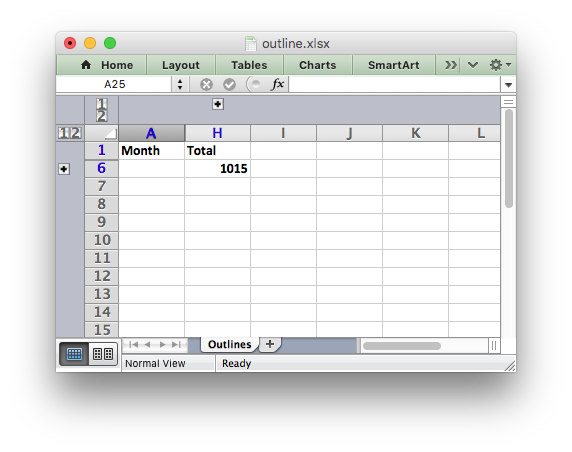Excel allows up to 7 outline levels. Therefore the `level` parameter should be in the range `0 <= level <= 7`.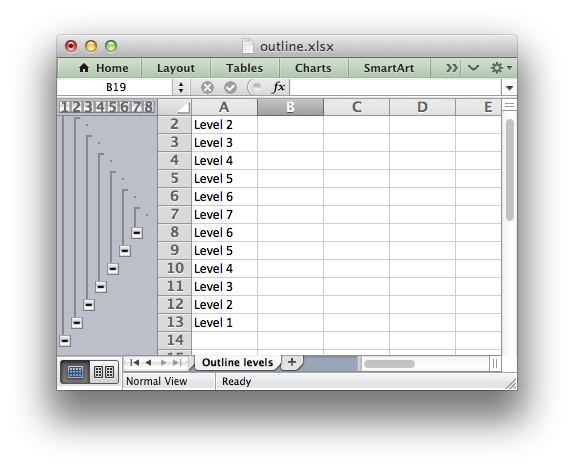Some additional outline properties can be set via the `worksheet_outline_settings()` worksheet function.

lxw_row_col_options
Options for rows and columns.
Definition: worksheet.h:839
LXW_DEF_ROW_HEIGHT
#define LXW_DEF_ROW_HEIGHT
Definition: worksheet.h:76
worksheet_set_row_opt
lxw_error worksheet_set_row_opt(lxw_worksheet *worksheet, lxw_row_t row, double height, lxw_format *format, lxw_row_col_options *options)
Set the properties for a row of cells.
COLS
#define COLS(cols)
Convert an Excel A:B column range into a (col1, col2) pair.
Definition: utility.h:63
worksheet_set_column_opt
lxw_error worksheet_set_column_opt(lxw_worksheet *worksheet, lxw_col_t first_col, lxw_col_t last_col, double width, lxw_format *format, lxw_row_col_options *options)
Set the properties for one or more columns of cells with options.
lxw_row_col_options::hidden
uint8_t hidden
Definition: worksheet.h:841
LXW_DEF_COL_WIDTH
#define LXW_DEF_COL_WIDTH
Definition: worksheet.h:73